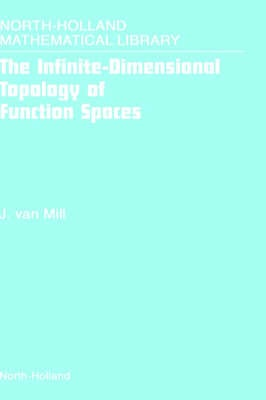•# The Infinite-Dimensional Topology of Function Spaces: Volume 64 - North-Holland Mathematical Library (Hardback)

(author)
£128.00
Hardback 642 Pages / Published: 15/06/2001
• We can order this

Usually dispatched within 15 working days

In this book we study function spaces of low Borel complexity. Techniques from general topology, infinite-dimensional topology, functional analysis and descriptive set theory are primarily used for the study of these spaces. The mix of methods from several disciplines makes the subject particularly interesting. Among other things, a complete and self-contained proof of the Dobrowolski-Marciszewski-Mogilski Theorem that all function spaces of low Borel complexity are topologically homeomorphic, is presented. In order to understand what is going on, a solid background in infinite-dimensional topology is needed. And for that a fair amount of knowledge of dimension theory as well as ANR theory is needed. The necessary material was partially covered in our previous book `Infinite-dimensional topology, prerequisites and introduction'. A selection of what was done there can be found here as well, but completely revised and at many places expanded with recent results. A `scenic' route has been chosen towards the Dobrowolski-Marciszewski-Mogilski Theorem, linking the results needed for its proof to interesting recent research developments in dimension theory and infinite-dimensional topology. The first five chapters of this book are intended as a text for graduate courses in topology. For a course in dimension theory, Chapters 2 and 3 and part of Chapter 1 should be covered. For a course in infinite-dimensional topology, Chapters 1, 4 and 5. In Chapter 6, which deals with function spaces, recent research results are discussed. It could also be used for a graduate course in topology but its flavor is more that of a research monograph than of a textbook; it is therefore more suitable as a text for a research seminar. The book consequently has the character of both textbook and a research monograph. In Chapters 1 through 5, unless stated otherwise, all spaces under discussion are separable and metrizable. In Chapter 6 results for more general classes of spaces are presented. In Appendix A for easy reference and some basic facts that are important in the book have been collected. The book is not intended as a basis for a course in topology; its purpose is to collect knowledge about general topology. The exercises in the book serve three purposes: 1) to test the reader's understanding of the material 2) to supply proofs of statements that are used in the text, but are not proven there 3) to provide additional information not covered by the text. Solutions to selected exercises have been included in Appendix B. These exercises are important or difficult.

Publisher: Elsevier Science & Technology
ISBN: 9780444505576
Number of pages: 642
Weight: 1140 g
Dimensions: 234 x 156 x 38 mm

MEDIA REVIEWS
We strongly recommend this book to mathematicians working in Cp-theory, infinite-dimensional topology, or dimension theory and also to students interested in these topics. Mathematical Reviews